# SAS/ETS Web Examples

## Estimating GARCH Models

Contents | SAS Program

# Overview

The generalized autoregressive conditional heteroscedasticity (GARCH) model of Bollerslev (1986) is an important type of time series model for heteroscedastic data. It explicitly models a time-varying conditional variance as a linear function of past squared residuals and of its past values. The GARCH process has been widely used to model economic and financial time-series data.

Many extensions of the simple GARCH model have been developed in the literature. This example illustrates estimation of variants of GARCH models using the AUTOREG and MODEL procedures, which include the

Please note that parameter restrictions implied in the GARCH type models are not discussed in this example. If estimated parameters do not satisfy the desired restrictions in a specific model, the BOUNDS or RESTRICT statement can be used to explicitly impose the restrictions in PROC MODEL.

For other examples of GARCH type models, see "Heteroscedastic Modeling of the Federal Funds Rate."

# Details

The data used in this example are generated with the SAS DATA step. The following code generates a simple GARCH model with normally distributed residuals.

   %let df = 7.5;
%let sig1 = 1;
%let sig2 = 0.1 ;
%let var2 = 2.5;
%let nobs = 1000 ;
%let nobs2 = 2000 ;
%let arch0 = 0.1 ;
%let arch1 = 0.2 ;
%let garch1 = 0.75 ;
%let intercept = 0.5 ;

data normal;
lu = &var2;
lh = &var2;
do i= -500  to &nobs ;
/* GARCH(1,1) with normally distributed residuals */
h = &arch0 + &arch1*lu**2 + &garch1*lh;
u = sqrt(h) * rannor(12345) ;
y = &intercept + u;
lu = u;
lh = h;
if i > 0 then output;
end;
run;


See the SAS program for more code that generates other types of GARCH models.

## Simple GARCH Model with Normally Distributed Residuals

The simple GARCH(p,q) model can be expressed as follows.

Let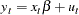The residual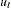is modeled as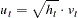where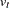is i.i.d. with zero mean and unit variance, and where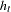is expressed as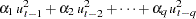In a standard GARCH model,is normally distributed. Alternative models can be specified by assuming different distributions for, for example, thedistribution, Cauchy distribution, etc.

To estimate a simple GARCH model, you can use the AUTOREG procedure. You use the GARCH= option to specify the GARCH model, and the (P= , Q= ) suboption to specify the orders of the GARCH model.

   proc autoreg data = normal ;
/* Estimate GARCH(1,1) with normally distributed residuals with AUTOREG*/
model y = / garch = ( q=1,p=1 ) ;
run ;
quit ;


The AUTOREG procedure produces the following output given in Figure 1.1 for a GARCH model with normally distributed errors .

Figure 1.1 Estimation Results using PROC AUTOREG
The AUTOREG Procedure

Variable DF Estimate Standard Error t Value Approx
Pr > |t|
Intercept 1 0.4783 0.0559 8.55 <.0001

Variable DF Estimate Standard Error t Value Approx
Pr > |t|
Intercept 1 0.4793 0.0323 14.86 <.0001
ARCH0 1 0.1159 0.0317 3.66 0.0003
ARCH1 1 0.2467 0.0389 6.35 <.0001
GARCH1 1 0.6972 0.0435 16.02 <.0001

You can also use the MODEL procedure to estimate a simple GARCH model. You must first specify the parameters in the model. Then specify the mean model and the variance model. The XLAG function returns the lag of the first argument if it is nonmissing. If the lag of the first argument is missing then the second argument is returned. The XLAG function makes it easy to specify the lag initialization for a GARCH process. The mse.y variable contains the value of the mean squared error for y at each iteration. These values are obtained automatically from first stage estimates, and are used to specify lagged values in estimation.

    /* Estimate GARCH(1,1) with normally distributed residuals with MODEL*/
proc model data = normal ;
parms arch0 .1 arch1 .2 garch1 .75 ;
/* mean model */
y = intercept ;
/* variance model */
h.y = arch0 + arch1*xlag(resid.y**2,mse.y) +
garch1*xlag(h.y,mse.y) ;
/* fit the model */
fit y / method = marquardt fiml ;
run ;
quit ;


Figure 1.2 shows the parameter estimates obtained by using the MODEL procedure.

Figure 1.2 Estimation Results using PROC MODEL
The MODEL Procedure

Nonlinear FIML Parameter Estimates
Parameter Estimate Approx Std Err t Value Approx
Pr > |t|
intercept 0.479341 0.0319 15.02 <.0001
arch0 0.115242 0.0345 3.34 0.0009
arch1 0.246811 0.0432 5.72 <.0001
garch1 0.697988 0.0494 14.13 <.0001

## GARCH Model with t-Distributed Residuals

To estimate a GARCH model with-distributed errors, you can use the AUTOREG procedure. You specify the GARCH(p,q) process with the GARCH=(p=,q=) option, and specify thedistributed error structure with the DIST= option.

    /* Estimate GARCH(1,1) with t-distributed residuals with AUTOREG*/
proc autoreg data = t ;
model y = / garch=( q=1, p=1 ) dist = t ;
run ;
quit;


The AUTOREG procedure produces the following output given in Figure 1.3 for a GARCH model with-distributed residuals.

Figure 1.3 Estimation Results using PROC AUTOREG
The AUTOREG Procedure

Variable DF Estimate Standard Error t Value Approx
Pr > |t|
Intercept 1 0.3997 0.0998 4.00 <.0001

Variable DF Estimate Standard Error t Value Approx
Pr > |t|
Variable Label
Intercept 1 0.5294 0.0374 14.14 <.0001
ARCH0 1 0.1244 0.0328 3.80 0.0001
ARCH1 1 0.2919 0.0309 9.44 <.0001
GARCH1 1 0.7419 0.0199 37.27 <.0001
TDFI 1 0.1351 0.0250 5.41 <.0001 Inverse of t DF

You can also use the MODEL procedure to estimate the GARCH model with-distributed residuals. Use the ERRORMODEL statement to specify the-distributed residuals. First specify the dependent variable name, a tilde(), and then the name of the error distribution with its parameters. The degrees of freedom for thedistribution are also estimated as a parameter in the MODEL procedure.

    /* Estimate GARCH(1,1) with t-distributed residuals with MODEL*/
proc model data = t ;
parms   df 7.5 arch0 .1 arch1 .2 garch1 .75 ;
/* mean model */
y = intercept ;
/* variance model */
h.y = arch0 + arch1 * xlag(resid.y **2, mse.y)  +
garch1*xlag(h.y, mse.y);
/* specify error distribution */
errormodel y ~ t(h.y,df);
/* fit the model */
fit y / method=marquardt;
run;
quit;


The MODEL procedure produces the following output given in Figure 1.4 for the GARCH model with-distributed errors.

Figure 1.4 Estimation Results using PROC MODEL
The MODEL Procedure

Nonlinear Liklhood Parameter Estimates
Parameter Estimate Approx Std Err t Value Approx
Pr > |t|
intercept 0.528964 0.0376 14.05 <.0001
df 7.468812 1.2402 6.02 <.0001
arch0 0.091711 0.0249 3.69 0.0002
arch1 0.215409 0.0248 8.69 <.0001
garch1 0.740497 0.0222 33.34 <.0001

Note that the MODEL procedure outputs the estimate of the degree of freedom for thedistribution, while the AUTOREG procedure outputs the reciprocal of the estimated degree of freedom.

## GARCH Model with Cauchy Distributed Residuals

You can also estimate a GARCH model with Cauchy-distributed errors in the MODEL procedure. The log likelihood function for GARCH with Cauchy-distributed residuals can be expressed as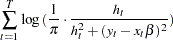We can then write the code using the model procedure.

   /* Estimate GARCH(1,1) with Cauchy distributed residuals */
proc model data = cauchy ;
parms arch0 .1 arch1 .2 garch1 .75 intercept .5 ;
mse_y = &var2 ;
/* mean model */
y = intercept ;
/* variance model */
h.y = arch0 + arch1 * xlag(resid.y ** 2, mse_y)  +
garch1 * xlag(h.y, mse_y);
/* specify error distribution */
obj = log(h.y/((h.y**2+resid.y**2) * constant('pi')));
obj = -obj ;
errormodel y ~ general(obj);
/* fit the model */
fit y / method=marquardt;
run;
quit;


The MODEL procedure produces the following output given in Figure 1.5 for a GARCH fit with Cauchy distributed residuals.

Figure 1.5 Estimation Results using PROC MODEL
The MODEL Procedure

Nonlinear Liklhood Parameter Estimates
Parameter Estimate Approx Std Err t Value Approx
Pr > |t|
intercept 0.499152 0.00385 129.73 <.0001
arch0 0.024821 0.00350 7.10 <.0001
arch1 0.005344 0.00180 2.97 0.0030
garch1 0.678644 0.0417 16.29 <.0001

## GARCH Model with Generalized Error Distribution Residuals

You can also estimate a GARCH model with GED (generalized error distribution) residuals with the MODEL procedure.

The log likelihood function for GARCH with GED residuals is expressed as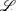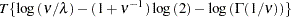whereis the sample size,is the gamma function,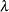is a constant given by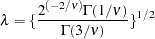and
is a positive parameter governing the thickness of the tails of the distribution. Note that for, constant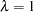, and the GED is the standard normal distribution. For more details about the generalized error distribution, see Hamilton (1994).

To estimate a GARCH model with GED residuals, you can use the following code.

   /* Estimate GARCH(1,1) with generalized error distribution residuals */
proc model data = normal ;
parms nu 2 arch0 .1 arch1 .2 garch1 .75;
control mse.y = &var2 ; /*defined in data generating step*/
/* mean model */
y = intercept ;
/* variance model */
h.y = arch0 + arch1 * xlag(resid.y ** 2, mse.y)  +
garch1 * xlag(h.y, mse.y);
/* specify error distribution */
lambda = sqrt(2**(-2/nu)*gamma(1/nu)/gamma(3/nu)) ;
obj = log(nu/lambda) -(1 + 1/nu)*log(2) - lgamma(1/nu)-
.5*abs(resid.y/lambda/sqrt(h.y))**nu - .5*log(h.y) ;
obj = -obj ;
errormodel y ~ general(obj,nu);
/* fit the model */
fit y / method=marquardt;
run;
quit;


The MODEL procedure produces the following output given in Figure 1.6 for a GARCH fit with residuals following a generalized error distribution.

Figure 1.6 Estimation Results using PROC MODEL
The MODEL Procedure

Nonlinear Liklhood Parameter Estimates
Parameter Estimate Approx Std Err t Value Approx
Pr > |t|
intercept 0.47119 0.0321 14.69 <.0001
nu 1.964288 0.1336 14.70 <.0001
arch0 0.172375 0.0359 4.80 <.0001
arch1 0.276887 0.0441 6.28 <.0001
garch1 0.637912 0.0476 13.39 <.0001

## GARCH-M Model

Another type of GARCH model is the GARCH-M model, which adds the heteroscedasticity term directly into the mean equation. In this example, consider the following specification: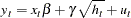The residualis modeled aswhereis i.i.d. with zero mean and unit variance, and whereis expressed asThe AUTOREG procedure enables you to specify the GARCH-M model with the MEAN= suboption of the GARCH= option. The MEAN= option specifies the functional form of the GARCH-M model. The values of the MEAN= option are

LINEAR, specifies the linear functionLOG, specifies the log functionSQRT, specifies the square-root functionIn this example, the square-root specification is considered.

   /* Estimate GARCH-M Model with PROC AUTOREG */
proc autoreg data= garchm ;
model y =  /  garch=( p=1, q=1,  mean = sqrt);
run;
quit;


The AUTOREG procedure produces the following output in Figure 1.7 for a GARCH-M model.

Figure 1.7 Estimation Results using PROC AUTOREG
The AUTOREG Procedure

Variable DF Estimate Standard Error t Value Approx
Pr > |t|
Intercept 1 1.2190 0.0480 25.39 <.0001

Variable DF Estimate Standard Error t Value Approx
Pr > |t|
Intercept 1 0.4987 0.1431 3.49 0.0005
ARCH0 1 0.0831 0.0275 3.02 0.0025
ARCH1 1 0.1695 0.0289 5.86 <.0001
GARCH1 1 0.7914 0.0321 24.62 <.0001
DELTA 1 0.5206 0.1196 4.35 <.0001

You can also use the MODEL procedure to estimate the GARCH-M model.

   /* Estimate GARCH-M Model with PROC MODEL */
proc model data = garchm ;
parms  arch0 .1 arch1 .2 garch1 .75 gamma .5 ;
h = arch0 + arch1*xlag(resid.y**2,mse.y) + garch1*xlag(h.y,mse.y);
y = intercept + gamma*sqrt(h) ;
h.y = h ;
fit y / fiml method = marquardt;
run;
quit;


This PROC MODEL step produces the following output as shown in Figure 1.8.

Figure 1.8 Estimation Results using PROC MODEL
The MODEL Procedure

Nonlinear FIML Parameter Estimates
Parameter Estimate Approx Std Err t Value Approx
Pr > |t|
arch0 0.082298 0.0268 3.07 0.0022
arch1 0.17043 0.0284 6.00 <.0001
garch1 0.79191 0.0318 24.87 <.0001
gamma 0.516689 0.1225 4.22 <.0001
intercept 0.502515 0.1488 3.38 0.0008

## EGARCH Model

The Exponential GARCH (EGARCH) model was proposed by Nelson (1991). It models the conditional variance ofas follows: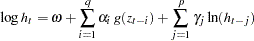where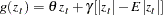The AUTOREG procedure also supports the EGARCH model. You can use the TYPE=EXP suboption of the GARCH= option to specify the EGARCH model.

   /* Estimate EGARCH Model with PROC AUTOREG */
proc autoreg data= egarch ;
model y =  / garch=( q=1, p=1 , type = exp) ;
run;
quit;


This produces the following output as shown in Figure 1.9.

Figure 1.9 Estimation Results using PROC AUTOREG
The AUTOREG Procedure

Variable DF Estimate Standard Error t Value Approx
Pr > |t|
Intercept 1 0.4790 0.0381 12.59 <.0001

Variable DF Estimate Standard Error t Value Approx
Pr > |t|
Intercept 1 0.4854 0.0361 13.43 <.0001
EARCH0 1 0.0960 0.0366 2.63 0.0087
EARCH1 1 0.2322 0.0744 3.12 0.0018
EGARCH1 1 0.7206 0.0961 7.50 <.0001
THETA 1 0.4403 0.1806 2.44 0.0148

You can also estimate the EGARCH model using the MODEL procedure.

   /* Estimate EGARCH Model with PROC MODEL */
proc model data = egarch ;
parms  earch0 .1 earch1 .2 egarch1 .75 theta .65 ;
/* mean model */
y = intercept ;
/* variance model */
if (_obs_ = 1 ) then
h.y = exp( earch0 + egarch1 * log(mse.y)  );
else h.y = exp(earch0 + earch1*zlag(g) + egarch1*log(zlag(h.y))) ;
g = theta*(-nresid.y) + abs(-nresid.y) - sqrt(2/constant('pi')) ;
/* fit the model */
fit y / fiml method = marquardt ;
run;
quit;


Note that in this example, the parameteris set to be equal to. The nresid.y variable gives the normalized residual of the variable y, i.e.,. Note also that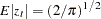if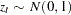.

The PROC MODEL code produces the following output for the EGARCH model as shown in Figure 1.10.

Figure 1.10 Estimation Results using PROC MODEL
The MODEL Procedure

Nonlinear FIML Parameter Estimates
Parameter Estimate Approx Std Err t Value Approx
Pr > |t|
intercept 0.485374 0.0368 13.18 <.0001
earch0 0.095713 0.0361 2.65 0.0081
earch1 0.233174 0.0690 3.38 0.0007
egarch1 0.721372 0.0934 7.73 <.0001
theta 0.434851 0.1790 2.43 0.0153

## QGARCH Model

The Quadratic GARCH model was proposed by Sentana (1995) to model asymmetric effects of positive and negative shocks.

A simple Quadratic GARCH(1,1) model describes the residual processaswhereis i.i.d. with zero mean and unit variance, and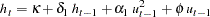whereis the asymmetric parameter that helps to separately identify the impact of positive and negative shocks on volatility.

The following code estimates a simple Quadratic GARCH(1,1) model in the MODEL procedure.

   /* Estimate Quadratic GARCH (QGARCH) Model */
proc model data = qgarch ;
parms arch0 .1 arch1 .2 garch1 .75 phi .2;
/* mean model */
y = intercept ;
/* variance model */
h.y = arch0 + arch1*xlag(resid.y**2,mse.y) + garch1*xlag(h.y,mse.y) +
phi*xlag(-resid.y,mse.y);
/* fit the model */
fit y / method = marquardt fiml ;
run ;
quit ;


Note that in specifying the equation for, you need to add a negative sign in front of the residual term, since PROC MODEL gives the negative of the residuals. This also applies to the GJR-GARCH model and the TGARCH model, to be discussed later in the example.

The code produces the following output shown in Figure 1.11 for a Quadratic GARCH(1,1) model.

Figure 1.11 Estimation Results using PROC MODEL
The MODEL Procedure

Nonlinear FIML Summary of Residual Errors
Equation DF Model DF Error SSE MSE Root MSE R-Square Adj R-Sq
y 5 995 2090.0 2.1005 1.4493 -0.0004 -0.0045
resid.y 995 996.5 1.0015 1.0007

Nonlinear FIML Parameter Estimates
Parameter Estimate Approx Std Err t Value Approx
Pr > |t|
intercept 0.510972 0.0356 14.37 <.0001
arch0 0.108659 0.0281 3.86 0.0001
arch1 0.166853 0.0294 5.67 <.0001
garch1 0.774571 0.0341 22.69 <.0001
phi 0.16391 0.0426 3.84 0.0001

## GJR-GARCH Model

Another asymmetric GARCH process is the GJR-GARCH model of Glosten, Jagannathan and Runkle (1993). They propose modeling, whereis i.i.d. with zero mean and unit variance, andwhereifandif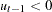.

You can use the following code to estimate a GJR-GARCH(1,1) model.

   /* Estimate GJR-GARCH Model */
proc model data = gjrgarch ;
parms arch0 .1 arch1 .2 garch1 .75 phi .1;
/* mean model */
y = intercept ;
/* variance model */
if zlag(resid.y) > 0 then
h.y = arch0 + arch1*xlag(resid.y**2,mse.y) + garch1*xlag(h.y,mse.y)  ;
else
h.y = arch0 + arch1*xlag(resid.y**2,mse.y) + garch1*xlag(h.y,mse.y) +
phi*xlag(resid.y**2,mse.y) ;
/* fit the model */
fit y / method = marquardt fiml ;
run ;
quit ;


This produces the following output shown in Figure 1.12 with the GJR-GARCH model.

Figure 1.12 Estimation Results using PROC MODEL
The MODEL Procedure

Nonlinear FIML Summary of Residual Errors
Equation DF Model DF Error SSE MSE Root MSE R-Square Adj R-Sq
y 5 995 13387.5 13.4548 3.6681 -0.0000 -0.0040
resid.y 995 996.2 1.0012 1.0006

Nonlinear FIML Parameter Estimates
Parameter Estimate Approx Std Err t Value Approx
Pr > |t|
intercept 0.557155 0.0513 10.85 <.0001
arch0 0.099621 0.0348 2.87 0.0042
arch1 0.185367 0.0386 4.80 <.0001
garch1 0.771999 0.0269 28.71 <.0001
phi 0.089578 0.0509 1.76 0.0788

## TGARCH Model

The Threshold GARCH model (TGARCH) of Zakoian (1994) is similar to the GJR GARCH, but it specifies the conditional standard deviation instead of conditional variance:where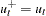if, and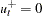if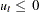. Similarly,if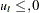, andif.

You can estimate TGARCH(1,1) model using the following code.

   /* Estimate Threshold Garch (TGARCH) Model */
proc model data = tgarch ;
parms arch0 .1 arch1_plus .1 arch1_minus .1 garch1 .75 ;
/* mean model */
y = intercept ;
/* variance model */
if zlag(resid.y) < 0 then
h.y = (arch0 + arch1_plus*zlag(-resid.y) + garch1*zlag(sqrt(h.y)))**2 ;
else
h.y = (arch0 + arch1_minus*zlag(-resid.y) + garch1*zlag(sqrt(h.y)))**2 ;
/* fit the model */
fit y / method = marquardt fiml ;
run ;
quit ;


This produces the following output for the TGARCH model as shown in Figure 1.13.

Figure 1.13 Estimation Results using PROC MODEL
The MODEL Procedure

Nonlinear FIML Parameter Estimates
Parameter Estimate Approx Std Err t Value Approx
Pr > |t|
intercept 0.504727 0.0124 40.57 <.0001
arch0 0.160549 0.0456 3.52 0.0004
arch1_plus 0.076379 0.0361 2.11 0.0347
arch1_minus 0.14484 0.0307 4.72 <.0001
garch1 0.632826 0.1161 5.45 <.0001

# References

Bollerslev, T. (1986), "Generalized Autoregressive Conditional Heteroskedasticity," Journal of Econometrics, 31, 307-327.

Glosten, L., Jagannathan, R. and Runkle, D. (1993), "On the Relation between the Expected Value and the Volatility of the Nominal Excess Return on Stocks," Journal of Finance, 48(5), 1779-1801.

Hamilton, J. D. (1994), Time Series Analysis, Princeton, NJ: Princeton University Press.

Nelson, B. (1991), "Conditional Heteroskedasticity in Asset Returns: A New Approach," Econometrica, 59, 347-370.

SAS Institute Inc. (1999), SAS/ETS User’s Guide, Version 8, Cary, NC: SAS Institute Inc.

Sentana, E. (1995), "Quadratic ARCH Models," Review of Economic Studies, 62, 639-661.

Zakoian, M. (1994), "Threshold Heteroscedastic Models," Journal of Economic Dynamics and Control, 18, 931-955.